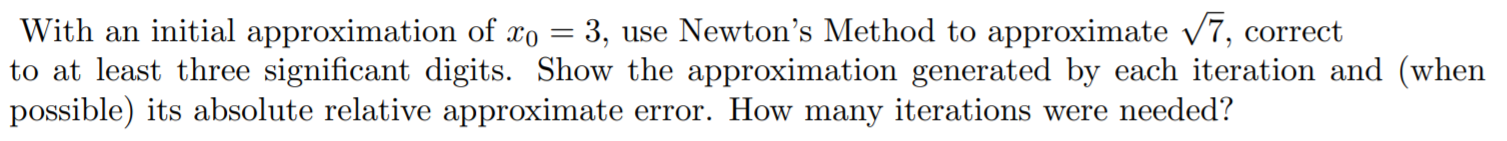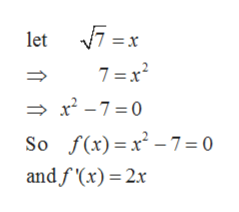# With an initial approximation of xo = 3, use Newton's Method to approximate /7, correctto at least three significant digits. Show the approximation generated by each iteration and (whenpossible) its absolute relative approximate error. How many iterations were needed?с

Question
5 viewshelp_outlineImage TranscriptioncloseWith an initial approximation of xo = 3, use Newton's Method to approximate /7, correct to at least three significant digits. Show the approximation generated by each iteration and (when possible) its absolute relative approximate error. How many iterations were needed? с fullscreen
check_circle

Step 1

Concept used: Newton’s Method If xn is an approximation of a solution of f(x)=0 and if f’(xn)≠0 then the next more accurate approximation is given by:

Step 2

To find the square root of 7 using newton’s method considering x be the square root of 7.
It gives:help_outlineImage Transcriptioncloselet 7 = x 7=x2 -7 0 So f(x) x-7 = 0 andfx) 2x fullscreen
Step 3

Taking the initial approximate x0=3

The first...

### Want to see the full answer?

See Solution

#### Want to see this answer and more?

Solutions are written by subject experts who are available 24/7. Questions are typically answered within 1 hour.*

See Solution
*Response times may vary by subject and question.
Tagged in

### Calculus